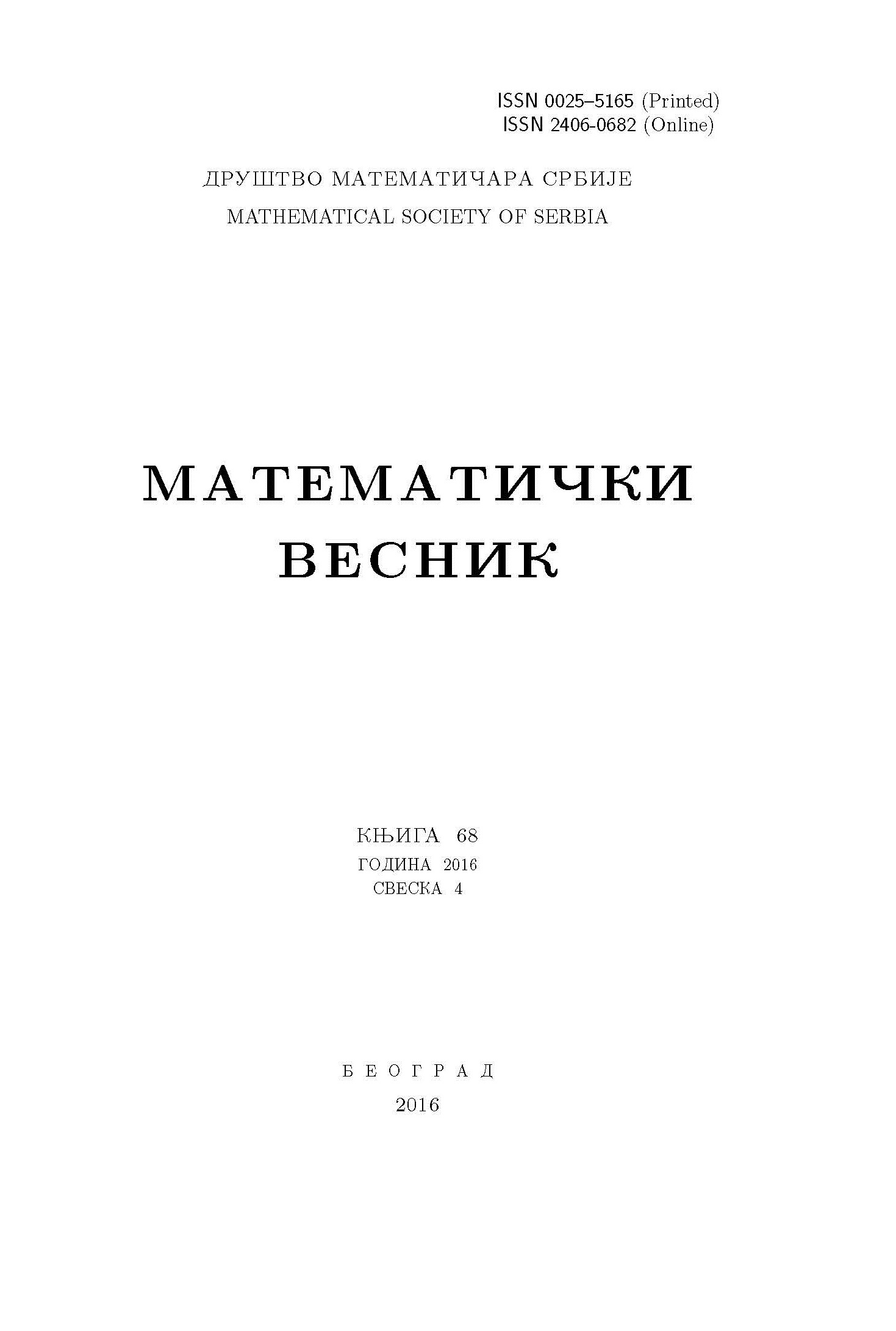﻿ Matematički Vesnik ﻿
 MATEMATIČKI VESNIK МАТЕМАТИЧКИ ВЕСНИКNORMALIZED LAPLACIAN ENERGY AND NORMALIZED LAPLACIAN-ENERGY-LIKE INVARIANT OF SOME DERIVED GRAPHS R. Amin, Sk. Md. Abu Nayeem AbstractFor a connected graph $G$, the smallest normalized Laplacian eigenvalue is 0 while all others are positive and the largest cannot exceed the value 2. The sum of absolute deviations of the eigenvalues from 1 is called the normalized Laplacian energy, denoted by $\mathbb{LE}(G)$. In analogy with Laplacian-energy-like invariant of $G$, we define here the normalized Laplacian-energy-like as the sum of square roots of normalized Laplacian eigenvalues of $G$, denoted by $\mathbb{LEL}(G)$.Keywords: Normalized Laplacian energy; normalized Laplacian-energy-like invariant; double graph; extended double cover; Mycielskian. MSC: 05C50 Pages:  229$-$241 Volume  74 ,  Issue  4 ,  2022

﻿